#Complex EigenvaluesConsider the linear homogeneous system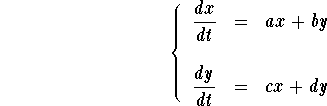The Characteristic polynomial is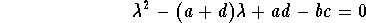In this section, we consider the case when the above quadratic equation has complex roots (that is if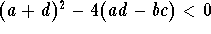). The roots (eigenvalues) are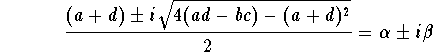where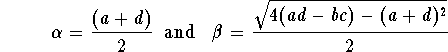In this case, the difficulty lies with the definition of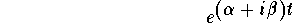In order to get around this difficulty we use Euler's formula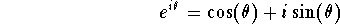Therefore, we have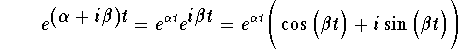In this case, the eigenvector associated to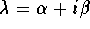will have complex components.

Example. Find the eigenvalues and eigenvectors of the matrix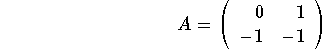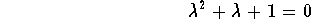Its roots are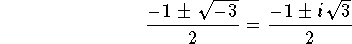Set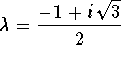. The associated eigenvector V is given by the equation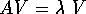. Set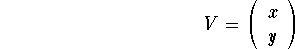The equationtranslates into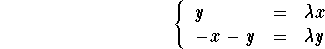Since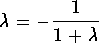, then the two equations are the same (which should have been expected, do you see why?). Hence we have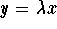which implies that an eigenvector is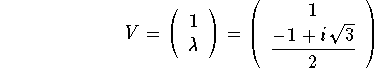We leave it to the reader to show that for the eigenvalue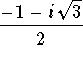, the eigenvector is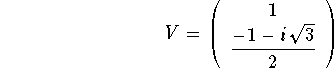Let us go back to the systemwith complex eigenvalues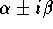. Note that if V, where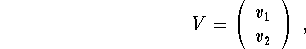is an eigenvector associated to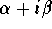, then the vector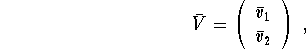(whereis the conjugate of v) is an eigenvector associated to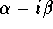. On the other hand, we have seen that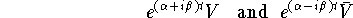are solutions. Note that these solutions are complex functions. In order to find real solutions, we used the above remarks. Set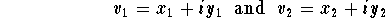then we have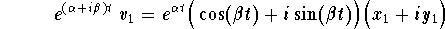which gives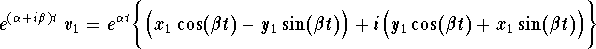Similarly we have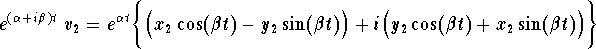Putting everything together we get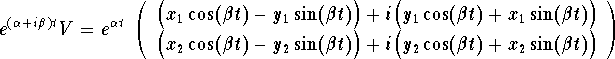Clearly this implies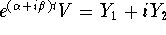where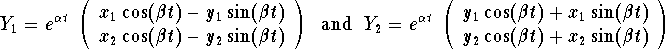It is easy to see that we have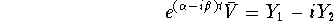Since the sum and difference of solutions lead to another solution, then both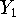and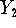are solutions of the system. These are real solutions. It is very easy to check in fact that they are linearly independent. Let us summarize the above technique.

Summary (of the complex case). Consider the systemWrite down the characteristic polynomialand find its roots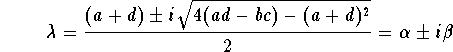we are assuming that. Note that at this step, you need to know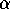and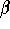. The common mistake is to forget to divide by 2.Find an eigenvector V associated to the eigenvalue. Write down the eigenvector as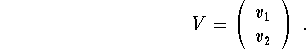Two linearly independent solutions are given by the formulasThe general solution is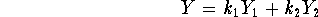where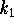and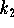are arbitrary numbers. Note that in this case, we have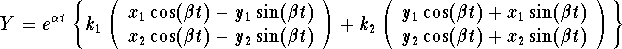Example. Consider the harmonic oscillator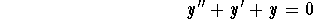Find the general solution using the system technique.

Answer. First we rewrite the second order equation into the system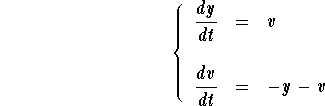The matrix coefficient of this system isWe have already found the eigenvalues and eigenvectors of this matrix. Indeed the eigenvalues are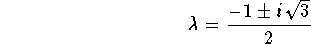Hence we have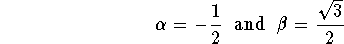The eigenvector associated tois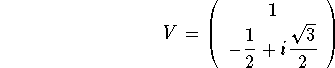Next we write down the two linearly independent solutions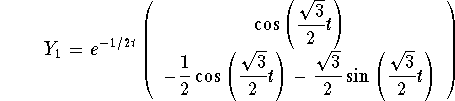and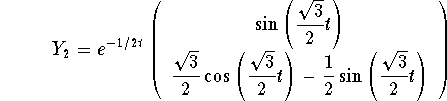The general solution of the equivalent system isor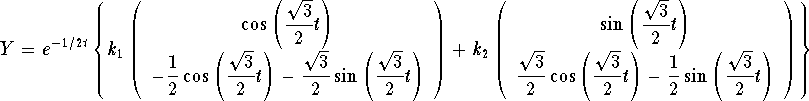Below we draw some solutions. Notice how the solutions spiral and dye at the origin (see the discussion below)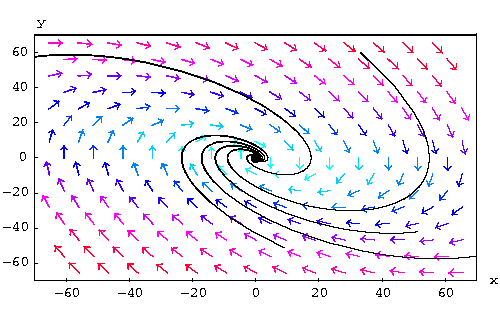Since we are looking for the general solution of the differential equation, we only consider the first component. Therefore we have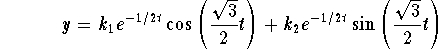You may want to check that the second component is just the derivative of y.
Below we draw some solutions for the differential equation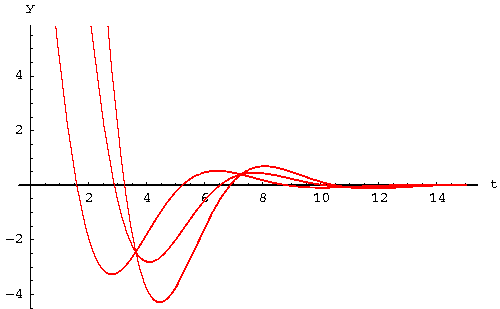Qualitative Analysis of Systems with Complex Eigenvalues.

Recall that in this case, the general solution is given byThe behavior of the solutions in the phase plane depends on the real part. Indeed, we have three cases:the case: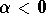. The solutions tend to the origin (when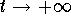) while spiraling. In this case, the equilibrium point is called a spiral sink.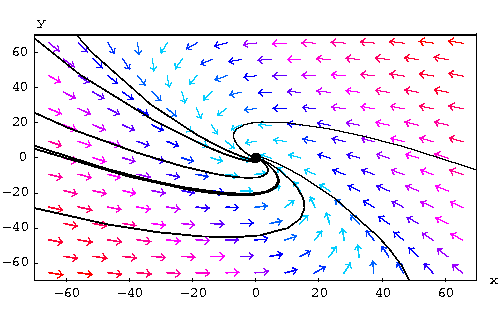The case: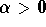The solutions explode or get away from the origin (when) while spiraling. In this case, the equilibrium point is called a spiral source.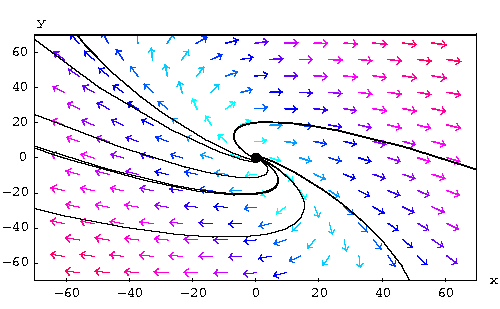The case: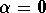The solutions are periodic. This means that the trajectories are closed curves or cycles. In this case, the equilibrium point is called a center.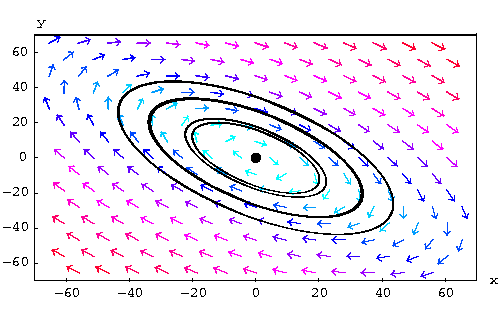### If you would like more practice, click on Example.[Differential Equations] [First Order D.E.]
[Geometry] [Algebra] [Trigonometry ]
[Calculus] [Complex Variables] [Matrix Algebra]S.O.S MATHematics home page

Do you need more help? Please post your question on our S.O.S. Mathematics CyberBoard.Author: Mohamed Amine Khamsi